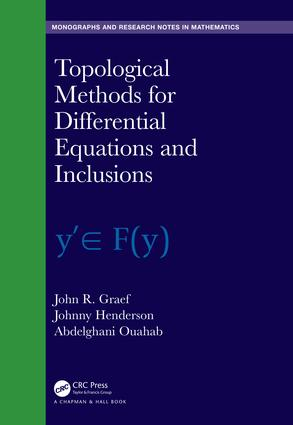Topological Methods for Differential Equations and Inclusions

1st Edition

CRC Press

360 pages

Hardback: 9781138332294
pub: 2018-10-02
\$159.95
x
eBook (VitalSource) : 9780429446740
pub: 2018-09-25
from \$28.98

FREE Standard Shipping!

Description

Topological Methods for Differential Equations and Inclusions covers the important topics involving topological methods in the theory of systems of differential equations. The equivalence between a control system and the corresponding differential inclusion is the central idea used to prove existence theorems in optimal control theory. Since the dynamics of economic, social, and biological systems are multi-valued, differential inclusions serve as natural models in macro systems with hysteresis.

Introduction

1 Background in Multi-valued Analysis

2 Hausdorff□-Pompeiu Metric Topology

3 Measurable Multifunctions

4 Continuous Selection Theorems

5 Linear Multivalued Operators

6 Fixed Point Theorems

7 Generalized Metric and Banach Spaces

8 Fixed Point Theorems in Vector Metric and Banach Spaces

9 Random □fixed point theorem

10 Semigroups

11 Systems of Impulsive Diff□erential Equations on the Half-line

12 Diff□erential Inclusions

13 Random Systems of Diff□erential Equations

14 Random Fractional Di□fferential Equations via Hadamard Fractional Derivative

15 Existence Theory for Systems of Discrete Equations

16 Discrete Inclusions

17 Semilinear System of Discrete Equations

18 Discrete Boundary Value Problems

19 Appendix

John R. Graef is professor of mathematics at the University of Tennessee at Chattanooga and previously was on the faculty at Mississippi State University.

Johnny Henderson is distinguished professor of mathematics at Baylor University. He also has held faculty positions at Auburn University and the Missouri University of Science and Technology. He is an Inaugural Fellow of the American Mathematical Society.

Abdelghani Ouahab is professor of Mathematics, Laboratory of Mathematics, Djilali-Liab\'es University Sidi Bel Abb\'es , Algeria

Each of the authors of this book has extensive research interests, including: boundary value problems for ordinary and functional differential equations and inclusions; difference equations; impulsive systems; fractional equations; dynamic equations on time scales; integral equations; boundary value problems; nonlinear oscillations, and applications to biological systems; functional differential equations and dynamic equations on time scales, along with extensions and generalizations involving multivalued analysis, and as well as in fractional analogues of those topics.